Courses

## 20 Questions MCQ Test Mathematics (Maths) Class 6 | Olympiad Test: Fractions

Description
This mock test of Olympiad Test: Fractions for Class 6 helps you for every Class 6 entrance exam. This contains 20 Multiple Choice Questions for Class 6 Olympiad Test: Fractions (mcq) to study with solutions a complete question bank. The solved questions answers in this Olympiad Test: Fractions quiz give you a good mix of easy questions and tough questions. Class 6 students definitely take this Olympiad Test: Fractions exercise for a better result in the exam. You can find other Olympiad Test: Fractions extra questions, long questions & short questions for Class 6 on EduRev as well by searching above.
QUESTION: 1

### Which option gives an equivalent fraction of 13/25

Solution: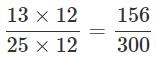QUESTION: 2

Solution:
QUESTION: 3

### What is the simplified form of the product 12/24 and 36/72

Solution:

12/24 x 36/72 = 1/2 x 1/2 = 1/4

QUESTION: 4

Give an example for a proper fraction.

Solution:

In a proper fraction, the numerator is less than the denominator.

QUESTION: 5

What is the fractional form of five eighteenths?

Solution:

The fractional form of five eighteenths

= 5/18

QUESTION: 6

Which fraction represents the shaded part of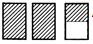?

Solution:

The shaded part represents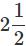QUESTION: 7

What are the fractions with the same denominator called?

Solution:

Like fractions have same denominator.

QUESTION: 8

Raju scored 9 marks in maths test. If the maximum marks of the test is 25, how is Raju's score represented as a fraction?

Solution:

Maximum marks = 25 Marks scored by Raju = 9

∴ Raju's score represented as a fraction

= 9/25

QUESTION: 9

Which of the following fractions is the largest?

Solution:

All fractions have the same numerator. So, the fraction having the smallest denominator is the largest.

∴ 29/23 is the required largest fraction.

QUESTION: 10

What is the value of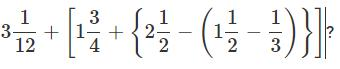Solution: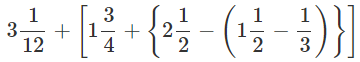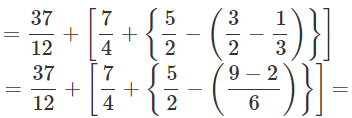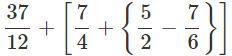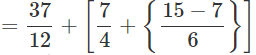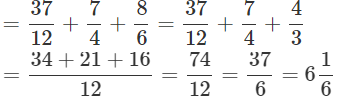QUESTION: 11

What should be added to 11/17 to make it 15/17?

Solution:

15/17 - 11/17 = 15 - 11/17 = 4/17

QUESTION: 12

By how much is 19/20 greater than 2/20?

Solution:

19/20 - 2/20  = 19 - 2/20 = 17/20

QUESTION: 13

What do you call fractions with different denominators?

Solution:

Unlike fractions have different denominators.

QUESTION: 14

What type of fraction is the sum 3/4 + 2/4?

Solution:

3/4 + 2/4 = 3+2/4 = 5/4 is an improper fraction. Since, the numerator is greater than the denominator.

QUESTION: 15

Which of these makes a whole?

Solution:
QUESTION: 16

In which of the following does the shaded part represent one fourth of its whole?

Solution:

(a) In the given object, there are a total of 4 squares and 1 square is shaded amongst them. Therefore, the ratio of shaded square to the total number of squares is:

which is one fourth of the whole object.

(b) In the given object, there are no equal parts which simply means that there is no one fourth of the whole object.

(c) In the given triangular object, there are no equal parts which simply means that there is no one fourth of the whole object.

(d) In the given object, there are a total of 3 squares and 1 square is shaded amongst them. Therefore, the ratio of shaded square to the total number of squares is:

which is not one fourth of the whole object.

QUESTION: 17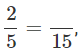What number should replace thein the given equation?

Solution:
QUESTION: 18

What are the fractions which have one as the numerator called?

Solution:

unit fraction is a rational number written as a fraction where the numerator is one and the denominator is a positive integer. A unit fraction is therefore the reciprocal of a positive integer, 1/n. Examples are 1/1, 1/2, 1/3, 1/4 ,1/5, etc.

QUESTION: 19

How is the fractional number for "3 out of 7 of the fruits are apples" written?

Solution:
QUESTION: 20

Two fractions are equivalent if their cross multiplications are

Solution: